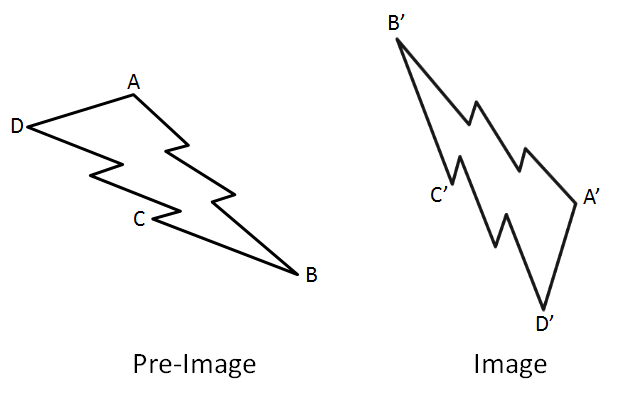# Transformations – Shortest Sequence

Directions: What is the fewest number of transformations needed to take pre-image ABCD to image A’B’C’D’?### Hint

What transformations do you need to use? Can you use the same transformations and do it another way?

A single reflection across a line that is roughly parallel to line segment DA in the pre-image will do it in one move. Otherwise students will likely get it in 2 moves by using a rotation and a reflection.

Source: Robert Kaplinsky

## Similar Triangles

Directions: Using the digits 0 to 9 at most one time each and as many …

1.A reflection that takes A to A’ can only be in the perpendicular bisector of AA’
Same goes for B and B’ and these two lines are not the same. So no single reflection.
Maybe they put in the wrong diagram?

•It means take ABCD to A’B’C’D’ up to translation; the actual location of the shapes is meant to be irrelevant here.

•Yes, Ben is correct.

2.Chelsea McClellan

I should state that the actual location is irrelevant then. Not stating that would create major confusion.

•Reflection between the middle of the pre-image and image, and rotate 180degree at the middle point.

3.Its kind of unclear what its asking, but if it means orientation then reflect across line AD

•2 transformations. Reflection and a rotation.

4.You could rotate the pre imagine until it looks like a mirror image of shape A’B’C’D’, then a reflection between the two images.

5.A reflection across the middle of the two shapes that’s vertical and then a rotation 90 degrees clockwise